# Signal Source Separation Using W-Net Architecture

This example shows how to separate two mixed signal sources using a deep learning network. Source separation is a common and complex signal processing problem that finds use in audio, vibration analysis, and biomedical applications. It consists of separating the signal components of a signal mixture when only the mixture is available.

An important source separation problem consists of discerning fetal and maternal electrocardiogram (ECG) signals present in noninvasive measurements taken on the abdominal area of a pregnant patient. This is an important problem because, if solved correctly, it can allow physicians to monitor the fetal ECG with minimum risk. Fetal cardiac monitoring and assessment during pregnancy are used for the early detection of fetal cardiac conditions.

This example uses simulated noninvasive abdominal ECG measurements on pregnant patients to illustrate how to solve the difficult problem of separating the fetal ECG and maternal ECG signals using a deep network. The source separation deep learning architecture used in this example is not limited to ECG signals and can be used in many other applications.

### FECGSYN Data Set

This example uses the FECGSYN PhysioNet data set , , which contains simulated adult and noninvasive fetal ECG signals. The data is generated using the FECGSYN simulator . The simulator represents maternal and fetal hearts as punctual dipoles with different magnitudes and spatial positions. It obtains fetal–maternal mixtures by treating each abdominal signal and noise component as an individual source whose signal is propagated onto the observational points (electrodes). This database is able to provide separate waveform files for each signal source, making it ideal to test a source separation deep learning model.

The FECGSYN consists of simulated ECG signals corresponding to ten different subjects. For each subject, simulations produced a fetal ECG (`fECG`), a maternal ECG (`mECG`), and two noise sources, all sampled at a rate of 250 Hz for five minutes. The original data set repeats simulations five times for five different SNR levels, for 34 ECG channels or "electrodes", and for five different measurement scenarios or cases. In this example we use a subset of the data set and consider all ten subjects, a single channel (channel 19 from the original data set), four SNR levels (3, 6, 9, and 12 dB), and three different measurement cases labeled C0, C1, and C3. As mentioned before, the simulation was repeated over five iterations for each combination of subject, SNR value, and measurement case, yielding a total of 10 subjects × 4 SNRs × 3 cases × 5 iterations = 600 files. There are three different measurement cases:

• Case 0 (C0) — Baseline ECG signals

• Case 1 (C1) — Fetal movement + C0

• Case 3 (C3) — Signals with varying maternal and fetal heart rates + Noise from uterine contractions

The data set contains one MAT-file for each combination of subject, SNR level, iteration, and measurement case. The filenames use the format Ij_Ck.mat, where `j` is the iteration number (1 to 5) and `k` is the measurement case identifier (0, 1, 3). Each MAT-file contains these variables:

• `mECG` — Maternal ECG signal

• `fECG` — Fetal ECG signal

• `mECG_QRS` — QRS peak locations for the maternal ECG signal as annotated by an expert system

• `fECG_QRS` — QRS peak locations for the fetal ECG signal as annotated by an expert system

• `noise1` — First noise source

• `noise2` — Second noise source

All signals have been bandpass filtered into the frequency range from 5 Hz to 90 Hz.

The abdominal ECG signal (`aECG`) for each file is computed as the following mixture:

`$\mathrm{aECG}=\mathrm{mECG}+\mathrm{fECG}+\mathrm{noise1}+\mathrm{noise2}$`

The `mECG_QRS` and `fECG_QRS` variables contain QRS peak locations of the maternal and fetal ECG signals and can be used to validate the efficacy of a source separation algorithm to identify correct heartbeat locations in time.

This example uses the data from the first nine subjects to train a deep network and the data from the tenth subject to test the network performance. The training data size is about 1.15 GB, and the training of the deep learning network takes a few hours even when run on a GPU. If you want to skip downloading the training data and the training process, set the `trainNetworkFlag` flag to `false`. If the flag is set to `false`, the example downloads a pretrained network that can be used to perform source separation on the test data. The example always downloads the test data corresponding to subject 10.

`trainNetworkFlag =true;`

Download the train and test data sets using the `downloadSupportFile` function. The data will be unzipped to the `tempdir` directory. If you want the data at a different location, change `trainingDatasetFolder` and `testDatasetFolder` to the desired locations.

```if trainNetworkFlag % Download training data set trainingDatasetZipFile = matlab.internal.examples.downloadSupportFile('SPT','data/fetal-ecg-source-separation-trainingData.zip'); trainingDatasetFolder = fullfile(tempdir,'fetal-ecg-source-separation-trainingData'); if ~exist(trainingDatasetFolder,'dir') unzip(trainingDatasetZipFile,trainingDatasetFolder); end end % Download test data set testDatasetZipFile = matlab.internal.examples.downloadSupportFile('SPT','data/fetal-ecg-source-separation-testData.zip'); testDatasetFolder = fullfile(tempdir,'fetal-ecg-source-separation-testData'); if ~exist(testDatasetFolder,'dir') unzip(testDatasetZipFile,testDatasetFolder); end```

Create a signal datastore to access the files in the test data set. Specify the names of the variables that you want the datastore to read from each file.

```testDS = signalDatastore(testDatasetFolder,IncludeSubfolders=true, ... SignalVariableNames=["mECG" "fECG" "noise1" "noise2" "mECG_QRS" "fECG_QRS"]);```

Plot the first 2048 samples of the ECG signals for case C1 and SNR of 3 dB. Overlay the annotated QRS peaks for each signal.

```idx = contains(testDS.Files,fullfile("snr03dB","I1_C1.mat")); sds3dBC1 = subset(testDS,idx); data = preview(sds3dBC1); [mECG,fECG,noise1,noise2,mECG_QRS,fECG_QRS] = data{:}; % Abdominal ECG mixture aECG = mECG(1:2048) + fECG(1:2048) + noise1(1:2048) + noise2(1:2048); figure subplot(3,1,1) plot(aECG) xline(fECG_QRS(1:50),":",Color="#77AC30") xline(mECG_QRS(1:50),":",Color="#D95319") axis([0 2048 -0.6 1]) title("aECG (red = mECG QRS peaks, green = fECG QRS peaks)") subplot(3,1,2) plot(fECG(1:2048)) xline(fECG_QRS(1:50),":",Color="#77AC30") axis([0 2048 -0.6 1]) title("fECG") subplot(3,1,3) plot(mECG(1:2048)) xline(mECG_QRS(1:50),":",Color="#D95319") axis([0 2048 -0.6 1]) title("mECG")```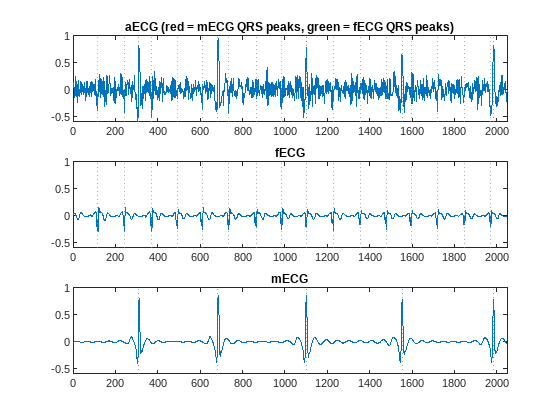Notice the large difference in scale between the `mECG` and `fECG` signals.

### Prepare Training Data

This example uses the data from the first nine subjects to train a deep network and the data from the tenth subject to test the network performance. To train the network, each signal is broken into segments of 1024 samples for a total of 73 segments per signal. Set up a signal datastore that reads the ECG signals and noise realizations for subjects 1 to 9. Transform the datastore to obtain `aECG`, `mECG`, and `fECG` signal segments of length 1024 samples. Each read to `trainDS` returns 73 segments of length 1024 `aECG`, `mECG`, and `mECG` signals formatted using `CBT` (channel-batch-time) dimensions. In addition to segmenting the signals into 1024-sample segments, the transform function, `getECGSegments`, also normalizes each segment using the `rescale` function to bring the signal levels to between –1 and 1. The rescaled segments are then centered using their median value.

```segmentLength = 1024; if trainNetworkFlag trainDS = signalDatastore(trainingDatasetFolder,IncludeSubfolders=true,SignalVariableNames=["mECG" "fECG" "noise1" "noise2"]); trainDS = transform(trainDS,@(d,f)getECGSegments(d,segmentLength)); end```

To speed up training, read all the training data into memory so that the signal segmentation and normalization happens only once. If you have a Parallel Computing Toolbox™ license, use the `UseParallel` parameter so that the read operations are done in parallel. Create an array datastore to iterate through the training signal segments.

```if trainNetworkFlag trainData = readall(trainDS,UseParallel=true); trainDS = arrayDatastore(trainData,OutputType="same"); end```

### W-Net Architecture for Source Separation

This example uses a so-called W-Net architecture to perform source separation . W-Net consists of two U-Net autoencoders  that have been modified to operate on 1-D signal inputs. A U-Net autoencoder is a deep network that encodes signal features reducing its size at each step and then decodes the features to recreate the original input signal. You can think of the encoder branch of the autoencoder as a feature extraction branch. The main idea of the W-Net architecture is to have one auto encoder to reproduce an `fECG` signal (`fECG` autoencoder) and another to reproduce an `mECG` signal (`mECG` autoencoder) when the input to the autoencoders is set to an `aECG` mixture. The connection between the two autoencoders happens in the encoding branches. You subtract the features obtained by the `mECG` autoencoder from the features obtained by the `fECG` autoencoder, effectively achieving separation of the `mECG` component from the `aECG` input and yielding the desired separated `fECG` signal. This figure shows the architecture in detail.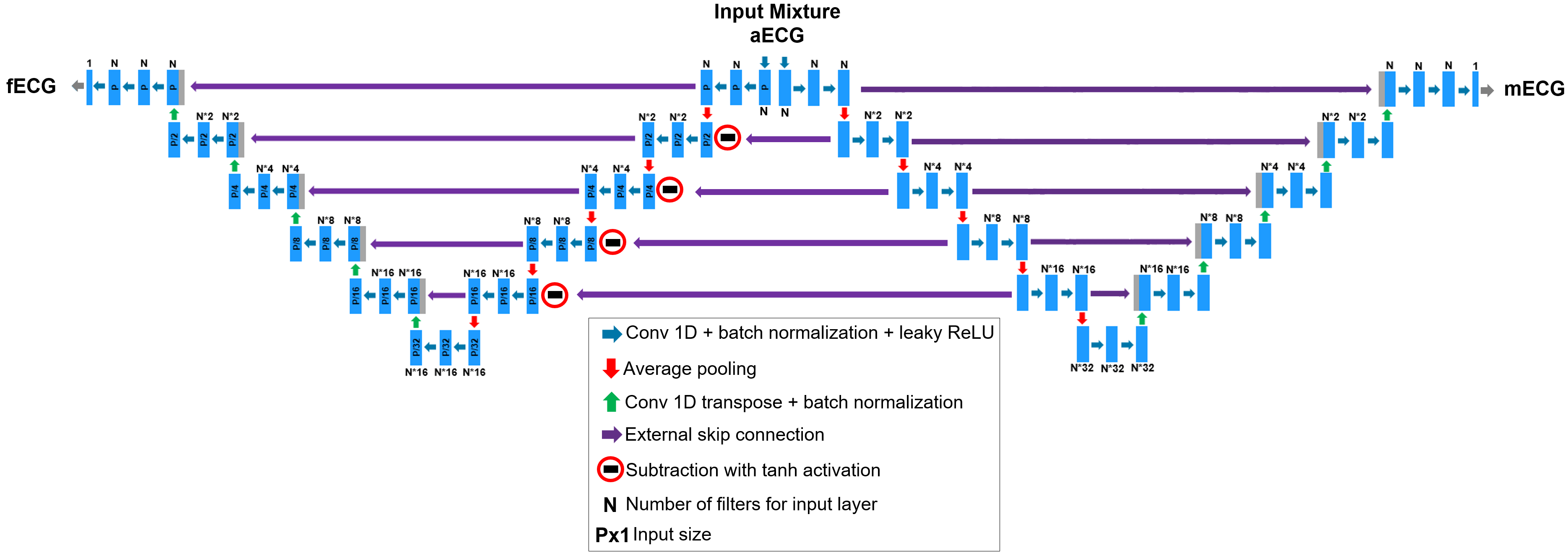Following reference , for the ECG source separation problem at hand set the filter size of the 1D convolutional layers to 4 for the `fECG` side and 35 for the `mECG` side. The number of filters used at the input 1D convolutional layers, `N` in the figure above, is set to `16`. The input size, `P` in the figure above, has already been described as 1024. Create the W-Net network architecture using the createWNet function.

```if trainNetworkFlag filterSize_fECG = 4; filterSize_mECG = 35; numFilters_fECG = 16; numFilters_mECG = 16; lgraph = createWNet(segmentLength,filterSize_fECG,numFilters_fECG,filterSize_mECG,numFilters_mECG); wNet = dlnetwork(lgraph); end```

### Training Loop

You need a training loop to train the W-Net model because you need to define a loss that combines the losses of the `fECG` and `mECG` branches of the network. The `modelLoss` function computes the training loss as the weighted sum of the mean absolute deviation between the actual and predicted ECG signals:

`$\mathrm{loss}=\mathrm{fECGWeight}×\mathrm{mean}\left(|\mathrm{fECGactual}-\mathrm{fECGpredicted}|\right)+\mathrm{mECGWeight}×\mathrm{mean}\left(|\mathrm{mECGactual}-\mathrm{mECGpredicted}|\right);$`

Set `fECGWeight` to a value greater than `mECGWeight` to reflect the fact that the primary signals of interest are the fetal ECGs.

Use an Adam optimizer to update the network learnable parameters and specify an initial learn rate, a decay factor, the number of epochs, and the mini-batch size. The `minibatchqueue` outputs `miniBatchSize` batches of `aECG`, `mECG`, and `fECG` signal segments.

Due to the large size of the data set, the training process may take several hours. If your machine has a GPU and Parallel Computing Toolbox™, set the `useGPUflag` flag to `true` to speed up the training process.

```useGPUflag =true; if trainNetworkFlag NumEpochs = 100; miniBatchSize = 512; learnRate = 0.0005; decay = 0.25; mECGWeight = 0.25; fECGWeight = 0.75; mbqTrain = minibatchqueue(trainDS, 3, ... MiniBatchSize=miniBatchSize,... MiniBatchFormat={'CBT','CBT','CBT'}, ... MiniBatchFcn=@processMB, ... DispatchInBackground=true); if useGPUflag mbqTrain.OutputEnvironment = "gpu"; end % Initialize some training loop variables trailingAvg = []; trailingAvgSq = []; iteration = 0; lossByIteration = 0; minLoss = Inf; % Loop over epochs and store the lowest loss network, reshuffle the % mini-batch queue at each epoch for epoch = 1:NumEpochs reset(mbqTrain) shuffle(mbqTrain) % Loop over mini-batches while hasdata(mbqTrain) iteration = iteration + 1; % Get the next mini-batch [aECGbatch,mECGbatch,fECGbatch] = next(mbqTrain); % Evaluate the model gradients and loss [loss,gradients,state] = dlfeval(@modelLoss,wNet,aECGbatch, ... mECGbatch,fECGbatch,mECGWeight,fECGWeight); lossByIteration(iteration) = loss; % Update the network state wNet.State = state; % Update the network parameters using an Adam optimizer [wNet,trailingAvg,trailingAvgSq] = adamupdate(wNet,gradients, ... trailingAvg,trailingAvgSq,iteration,learnRate,decay); end if loss < minLoss minLoss = loss; bestModel = wNet; % Uncomment the line below to save the best model so far %save Model.mat wNet end end wNet = bestModel; % Plot the loss by iteration figure plot(1:iteration,mag2db(lossByIteration)) grid on title("Training Loss by Iteration") xlabel("Iteration") ylabel("Loss (dB)") axis tight end```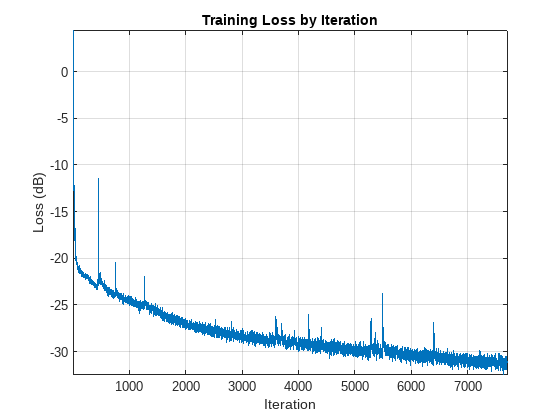Load a pretrained model if `trainNetworkFlag` is `false`. The model file will be unzipped to the `tempdir` directory. If you want the model at a different location, change `modelFolder` to the desired value.

```if ~trainNetworkFlag % Download the pre-trained network modelZipFile = matlab.internal.examples.downloadSupportFile('SPT','data/fetal-ecg-source-separation-model.zip'); modelFolder = fullfile(tempdir,'fetal-ecg-source-separation-model'); if ~exist(modelFolder,'dir') unzip(modelZipFile,modelFolder); end modelFile = fullfile(modelFolder,'fetal-ecg-source-separation-model','Model.mat'); load(modelFile) end```

### Test Model

To test the trained network, use the previously created test datastore, `testDS`, that points to data from subject 10. This datastore reads the ECG data and the QRS peak location annotations so they can be used to validate the predicted `mECG` and `fECG` signals. As was done for the training datastore, transform the test datastore to get segmented and normalized `aECG`, `mECG`, and `fECG` signals.

`testDS = transform(testDS,@(d,f)getECGSegments(d,segmentLength));`

Call the predict method of the trained network to get separated `mECG` and `fECG` signals from an `aECG` input. Take for example iteration 3 of case C1 with and SNR of 9 dB. Estimate the fetal and maternal waveforms for that case as follows.

```idx = contains(string(testDS.UnderlyingDatastores{1}.Files),fullfile("snr09dB","I3_C1.mat")); ds = subset(testDS,idx); data = read(ds); mECG_QRS = data(:,4); fECG_QRS = data(:,5); [aECGbatch,mECGbatch,fECGbatch] = processMB(data(:,1),data(:,2),data(:,3)); % Move the aECGbatch into a dlarray and call the predict method of the % trained network to estimate the source signals dlaECG = dlarray(aECGbatch,"CBT"); [dlpred_fECG,dlpred_mECG] = predict(wNet,dlaECG); pred_fECG = squeeze(extractdata(dlpred_fECG))'; pred_mECG = squeeze(extractdata(dlpred_mECG))'; pred_fECG = pred_fECG(:); pred_mECG = pred_mECG(:);```

Plot a few samples of the predicted waveforms. Overlay the annotated true QRS peaks using dotted lines.

```figure subplot(2,1,1) plot(pred_fECG(1:2048)) xline([fECG_QRS{1}; fECG_QRS{2}],":k") title("Predicted fECG") axis([1 2048 -1.5 1]) subplot(2,1,2) plot(pred_mECG(1:2048)) xline([mECG_QRS{1}; mECG_QRS{2}],":k") title("Predicted mECG") axis([1 2048 -1 2])```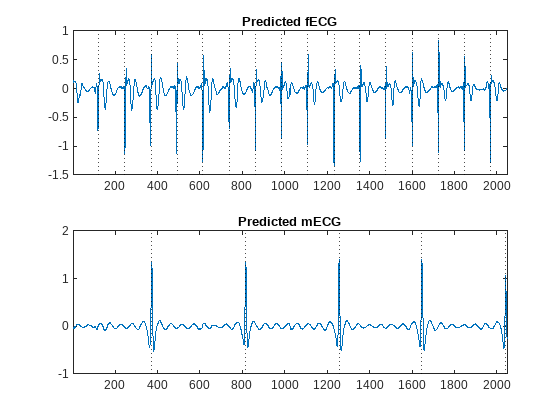Plot predicted ECG signals for the case of high (12 dB) and low (3 dB) SNRs, for iteration 4 measurements, and for all three measurement cases using the `plotPredictedECGs` function. `N` and `M` can be set to plot segments `N` to `N`+`M` for the case at hand.

```% Plot segment 4 for each case N = 4; M = 1; plotPredictedECGs(wNet,testDS,"12","C0","I4",N,M)````plotPredictedECGs(wNet,testDS,"12","C1","I4",N,M)``plotPredictedECGs(wNet,testDS,"12","C3","I4",N,M)`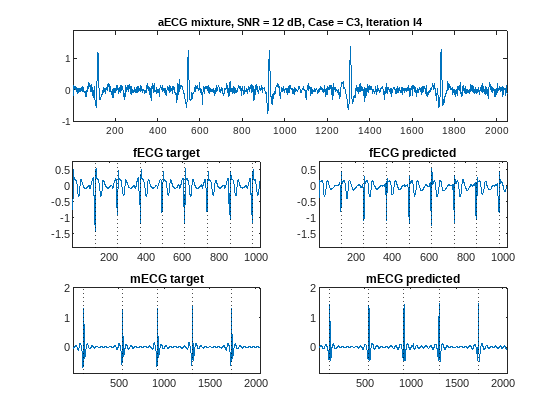`plotPredictedECGs(wNet,testDS,"03","C0","I4",N,M)`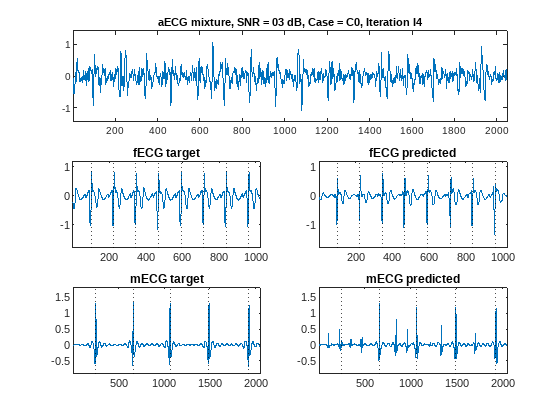`plotPredictedECGs(wNet,testDS,"03","C1","I4",N,M)`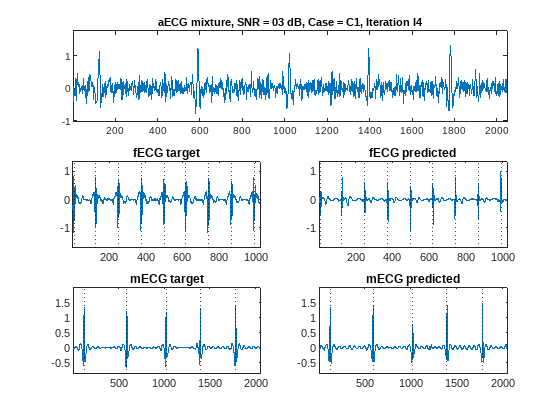`plotPredictedECGs(wNet,testDS,"03","C3","I4",N,M)``fECG` signals have faster heart rates than `mECGs` so we show fewer `fECG` points just for better visualization. The dotted lines on the plots correspond to annotated ground truth QRS peak locations. Proper location of QRS peaks is as important as the estimation of the overall signal shape. QRS peak locations allow estimation of heart rate and conditions like arrhythmia. Proper peak location should be considered when evaluating the performance of the source separation procedure.

Recall that the main purpose of this network is to extract fetal ECG signals, which are the most difficult to obtain from the mixture. In the W-Net architecture the primary target is the one estimated by the left U-Net branch, which corresponds to the first output of the network built in this example using the `modelLoss` function. Overall, the network does a very good job in estimating QRS peak locations and waveform shapes for both high- and low-SNR cases and different measurement conditions.

There are extreme measurement cases where the combination of noise, fetal movements, and heart rate variations are too severe for the network. For example, plot the ECG estimates for an SNR of 6 dB, measurement case C3, and iteration 5. In this case, the network fails to predict an acceptable `fECG` waveform.

`plotPredictedECGs(wNet,testDS,"06","C3","I5",N,M)`Plot the mean absolute deviation of the estimated `fECG` and `mECG` signals for all measurements of subject 10 using the `computeErrorsForAllCases` function.

`computeErrorsForAllCases(wNet,testDS)`The errors do not decrease monotonically with SNR because of the variability of all the different combinations of noise, fetal movement, and heart-rate irregularities.

### Conclusion

This example implements a W-Net architecture suitable for source separation of a mixture of two signals. The example analyzes the performance of the network using synthetic signal mixtures comprised of fetal and maternal ECG waveforms. The example shows that, in most scenarios, the network does a good job separating ECG signals and estimating correct waveform shapes and QRS peak locations.

### References

 Goldberger, Ary L., Luis A. N. Amaral, Leon Glass, Jeffrey M. Hausdorff, Plamen Ch. Ivanov, Roger G. Mark, Joseph E. Mietus, George B. Moody, Chung-Kang Peng, and H. Eugene Stanley. “PhysioBank, PhysioToolkit, and PhysioNet.” Circulation 101, no. 23 (June 13, 2000): e215–20. https://doi.org/10.1161/01.CIR.101.23.e215.

 F. Andreotti, J. Behar, and G. D. Clifford. Fetal ECG Synthetic Database v1.0.0 (physionet.org), April 29, 2016, Version 1.0.0.

 F. Andreotti, J. Behar, S. Zaunseder, J. Oster, and G. D. Clifford. "An Open-Source Framework for Stress-Testing Non-Invasive Foetal ECG Extraction Algorithms." Physiological Measurement, Volume 37, Number 5, 2016.

 K. J. Lee and B. Lee, "End-to-End Deep Learning Architecture for Separating Maternal and Fetal ECGs Using W-Net," IEEE Access, Volume 10, pp. 39782-39788, 2022.

 O. Ronneberger, P. Fischer, and T. Brox. "U-Net: Convolutional Networks for Biomedical Image Segmentation", MICCAI, May 18, 2015.

### Appendix: Helper Functions

The functions listed in this section are only for use in this example. They may change or be removed in a future release.

#### getECGSegments

This function creates `aECG` mixtures from `mECG`, `fECG`, and noise signals. The function breaks the ECG signals into segments of length `segmentLength`. Each segment is normalized and reshaped to a `CBT` format with `C` and `B` equal to 1. When the input to the function contains QRS peak locations, the function breaks the locations according to the start and end index of each segment.

```function outputCell = getECGSegments(cellInput,segmentLength) mECG = cellInput{1}; fECG = cellInput{2}; noise1 = cellInput{3}; noise2 = cellInput{4}; aECG = mECG + fECG + noise1 + noise2; % Segment the data and keep indices so that we can also segment the QRS % peak locations [idxs,~] = buffer(1:size(mECG,1),segmentLength); mECG = single(mECG(idxs)'); fECG = single(fECG(idxs)'); aECG = single(aECG(idxs)'); % Normalize for idx = 1:size(mECG,1) mECG(idx,:) = rescale(mECG(idx,:),-1,1); mECG(idx,:) = mECG(idx,:) - median(mECG(idx,:)); fECG(idx,:) = rescale(fECG(idx,:),-1,1); fECG(idx,:) = fECG(idx,:) - median(fECG(idx,:)); aECG(idx,:) = rescale(aECG(idx,:),-1,1); aECG(idx,:) = aECG(idx,:) - median(aECG(idx,:)); end numRows = size(mECG,1); % CBT format C=1 B=numRows T=segmentLength mECG = reshape(mECG,1,numRows,[]); fECG = reshape(fECG,1,numRows,[]); aECG = reshape(aECG,1,numRows,[]); % Create cell array with individual elements --> CBT format C=1 B=1 T=segmentLength mECGCell = mat2cell(mECG,1,ones(numRows,1),segmentLength)'; fECGCell = mat2cell(fECG,1,ones(numRows,1),segmentLength)'; aECGCell = mat2cell(aECG,1,ones(numRows,1),segmentLength)'; outputCell = [aECGCell mECGCell fECGCell]; if numel(cellInput) == 6 mECG_QRSTmp = cellInput{5}; fECG_QRSTmp = cellInput{6}; segmentLimits = [idxs(1,:)' idxs(end,:)']; numSegments = size(segmentLimits,1); mECG_QRS = cell(numSegments,1); fECG_QRS = cell(numSegments,1); for idx = 1:numSegments mECG_QRS{idx} = mECG_QRSTmp(mECG_QRSTmp >= segmentLimits(idx,1) & ... mECG_QRSTmp <= segmentLimits(idx,2)); fECG_QRS{idx} = fECG_QRSTmp(fECG_QRSTmp >= segmentLimits(idx,1) & ... fECG_QRSTmp <= segmentLimits(idx,2)); end outputCell = [outputCell mECG_QRS fECG_QRS]; end end```

#### processMB

This function converts cell array inputs, containing ECG segments, to mini-batches with `CBT` format.

```function [aECGbatch,mECGbatch,fECGbatch] = processMB(aECGCell,mECGCell,fECGCell) aECGbatch = cat(2,aECGCell{:}); mECGbatch = cat(2,mECGCell{:}); fECGbatch = cat(2,fECGCell{:}); end ```

#### modelLoss

This function feeds an `aECG` input to the network and computes the gradient and resulting loss.

```function [loss,grads,state] = modelLoss(net,aECG,mECG,fECG,mECGWeight,fECGWeight) [fECGpred,mECGpred,state] = net.forward(aECG); loss = stripdims(fECGWeight*mean(abs(fECG-fECGpred),"all") + ... mECGWeight*mean(abs(mECG-mECGpred),"all")); grads = dlgradient(loss,net.Learnables); loss = double(gather(extractdata(loss))); end```

#### plotPredictedECGs

This function plots actual and predicted ECG signals for a specified measurement case, iteration, and SNR value. The function plots segments `N` to `N`+`M`.

```function plotPredictedECGs(wNet,testDS,SNRstr,caseStr,iterStr,N,M) % testDS is datastore pointing to test data % SNRstr can be "12", "09", "06", "03" % iterStr can be "I1", "I2", "I3", "I4", "I5" % caseStr can be "C0", "C1", "C3" dataIdx = N:N+M; % Get a datastore with the requested case idx = contains(string(testDS.UnderlyingDatastores{1}.Files), ... fullfile("snr"+SNRstr+"dB",iterStr+"_"+caseStr+".mat")); ds = subset(testDS,idx); data = read(ds); data = data(dataIdx,:); mECG_QRS = data(:,4); fECG_QRS = data(:,5); [aECGbatch,mECGbatch,fECGbatch] = processMB(data(:,1),data(:,2),data(:,3)); % Move the aECGbatch into a dlarray and call the predict method of the % trained network to estimate the source signals dlaECG = dlarray(aECGbatch,"CBT"); [dlpred_fECG,dlpred_mECG] = predict(wNet,dlaECG); pred_fECG = extractdata(dlpred_fECG); pred_mECG = extractdata(dlpred_mECG); % Plot the results aECG = squeeze(aECGbatch)'; mECG = squeeze(mECGbatch)'; fECG = squeeze(fECGbatch)'; pred_fECG = squeeze(pred_fECG)'; pred_mECG = squeeze(pred_mECG)'; aECG = aECG(:); mECG = mECG(:); fECG = fECG(:); pred_mECG = pred_mECG(:); pred_fECG = pred_fECG(:); mECG_QRS = cat(1,mECG_QRS{:}); fECG_QRS = cat(1,fECG_QRS{:}); mECG_QRS = mECG_QRS - ((N-1)*1024-1) - 1; fECG_QRS = fECG_QRS - ((N-1)*1024-1) - 1; titleStr = "SNR = "+SNRstr+" dB, Case = "+caseStr+", Iteration "+iterStr; figure subplot(3,2,[1 2]) plot(aECG) title("aECG mixture, "+titleStr) minECG = min(aECG); maxECG = max(aECG); axis([1 length(aECG) minECG-abs(minECG*0.35) maxECG+maxECG*0.35]) minfECG = min(fECG); maxfECG = max(fECG); minPredfECG = gather(min(pred_fECG)); maxPredfECG = gather(max(pred_fECG)); minECG = min(minfECG,minPredfECG); maxECG = max(maxfECG,maxPredfECG); subplot(3,2,3) plot(fECG) xline(fECG_QRS,":k") title("fECG target") axis([1 floor(length(fECG)/2) minECG-abs(minECG*0.35) maxECG+maxECG*0.35]) subplot(3,2,4) plot(pred_fECG) xline(fECG_QRS,":k") title("fECG predicted") axis([1 floor(length(pred_fECG)/2) minECG-abs(minECG*0.35) maxECG+maxECG*0.35]) minmECG = min(mECG); maxmECG = max(mECG); minPredmECG = gather(min(pred_mECG)); maxPredmECG = gather(max(pred_mECG)); minECG = min(minmECG,minPredmECG); maxECG = max(maxmECG,maxPredmECG); subplot(3,2,5) plot(mECG) xline(mECG_QRS,":k") title("mECG target") axis([1 length(mECG) minECG-abs(minECG*0.35) maxECG+maxECG*0.35]) subplot(3,2,6) plot(pred_mECG) xline(mECG_QRS,":k") title("mECG predicted") axis([1 length(pred_mECG) minECG-abs(minECG*0.35) maxECG+maxECG*0.35]) end```

#### computeErrorsForAllCases

This function computes the mean absolute error between actual `fECG` and `mECG` signals and predicted ones for all SNR values, measurement cases, and iterations of subject 10.

```function computeErrorsForAllCases(wNet,testDS) % testDS is datastore pointing to test data of subject 10 SNRVect = ["03" "06" "09" "12"]; caseVect = ["C0" "C1" "C3"]; for SNRidx = 1:numel(SNRVect) SNRstr = SNRVect(SNRidx); for caseIdx = 1:numel(caseVect) caseStr = caseVect(caseIdx); idx = contains(string(testDS.UnderlyingDatastores{1}.Files),fullfile("snr"+SNRstr+"dB","I1_"+caseStr+".mat")); idx = idx | contains(string(testDS.UnderlyingDatastores{1}.Files),fullfile("snr"+SNRstr+"dB","I2_"+caseStr+".mat")); idx = idx | contains(string(testDS.UnderlyingDatastores{1}.Files),fullfile("snr"+SNRstr+"dB","I3_"+caseStr+".mat")); idx = idx | contains(string(testDS.UnderlyingDatastores{1}.Files),fullfile("snr"+SNRstr+"dB","I4_"+caseStr+".mat")); idx = idx | contains(string(testDS.UnderlyingDatastores{1}.Files),fullfile("snr"+SNRstr+"dB","I5_"+caseStr+".mat")); ds = subset(testDS,idx); data = readall(ds); [aECGbatch,mECGbatch,fECGbatch] = processMB(data(:,1),data(:,2),data(:,3)); dlaECG = dlarray(aECGbatch,"CBT"); [dlpred_fECG,dlpred_mECG] = predict(wNet,dlaECG); pred_mECG = extractdata(dlpred_mECG); pred_fECG = extractdata(dlpred_fECG); errMtx(caseIdx,SNRidx) = 0.5*mean(abs(mECGbatch - pred_mECG),'all') + 0.5*mean(abs(fECGbatch - pred_fECG),'all'); errMtxFecg(caseIdx,SNRidx) = mean(abs(fECGbatch - pred_fECG),'all'); errMtxMecg(caseIdx,SNRidx) = mean(abs(mECGbatch - pred_mECG),'all'); end end figure subplot(2,1,1) plot([3 6 9 12],errMtxFecg'); title("fECG mean absolute errors") xlabel("SNR") ylabel("MAE") legend("C0","C1","C3") grid on axis tight subplot(2,1,2) plot([3 6 9 12],errMtxMecg'); title("mECG mean absolute errors") xlabel("SNR") ylabel("MAE") legend("C0","C1","C3") grid on axis tight end```

#### createWNet

This function implements a W-Net architecture and returns a layer graph.

```function lgraph = createWNet(inputSize,filterSizeLeft,numFiltersLeft,filterSizeRight,numFiltersRight) lgraph = layerGraph; inputLayer = sequenceInputLayer(1,MinLength=inputSize,Name="inputMixture"); lgraph = addLayers(lgraph,inputLayer); % Define left and right U-Net branches % Layer name conventions - left means it belongs to left U-Net % - ds means down sample, us means upsample branch, % bridge is the final row in the autoencoder % - i_j means ith row, jth layer % Add left branch U-Net lgraph = createUNet(lgraph,filterSizeLeft,numFiltersLeft,"left"); lgraph = connectLayers(lgraph,'inputMixture','conv1d_left_ds_1_1'); % Add right branch U-Net lgraph = createUNet(lgraph,filterSizeRight,numFiltersRight,"right"); lgraph = connectLayers(lgraph,'inputMixture','conv1d_right_ds_1_1'); % Connect right U-Net encoder branch to subtraction layers lgraph = connectLayers(lgraph,"avgpool1d_right_1_to_2","subtraction_2/in2"); lgraph = connectLayers(lgraph,"avgpool1d_right_2_to_3","subtraction_3/in2"); lgraph = connectLayers(lgraph,"avgpool1d_right_3_to_4","subtraction_4/in2"); lgraph = connectLayers(lgraph,"avgpool1d_right_4_to_5","subtraction_5/in2"); end```

#### createUNet

This function implements the left and right U-Net branches needed to build a W-Net architecture.

```function lgraph = createUNet(lgraph,filterSize,numFilters,branchStr) % branchStr can be "left" or "right" % % Layer name conventions - left means it belongs to left U-Net % - ds means down sample, us means upsample branch % - i_j means ith row, jth layer numFiltScale = 1 + double(branchStr == "right"); if branchStr == "left" branchStrOutput = "outputLayer_left_targetSignal"; else branchStrOutput = "outputLayer_right_secondarySignal"; end unet = [ % Row 1 encoder branch convolution1dLayer(filterSize, numFilters, Padding="same", Name="conv1d_"+branchStr+"_ds_1_1") batchNormalizationLayer(Name="batchnorm_"+branchStr+"_ds_1_1") leakyReluLayer(0.01,Name="leakyrelu_"+branchStr+"_ds_1_1") convolution1dLayer(filterSize, numFilters, Padding="same", Name="conv1d_"+branchStr+"_ds_1_2") batchNormalizationLayer(Name="batchnorm_"+branchStr+"_ds_1_2") leakyReluLayer(0.01,Name="leakyrelu_"+branchStr+"_ds_1_2") convolution1dLayer(filterSize, numFilters, Padding="same",Name="conv1d_"+branchStr+"_ds_1_3") batchNormalizationLayer("Name","batchnorm_"+branchStr+"_ds_1_3") leakyReluLayer(0.01,"Name","leakyrelu_"+branchStr+"_ds_1_3") averagePooling1dLayer(2, Padding="same", Stride=2, Name="avgpool1d_"+branchStr+"_1_to_2") ]; % Row 2 encoder branch if branchStr == "left" unet = [unet functionLayer(@minus,NumInputs=2,Formattable=true,Acceleratable=true,Name="subtraction_2"); tanhLayer(Name="tanh_2") ]; end unet = [unet convolution1dLayer(filterSize, numFilters*2, Padding="same", Name="conv1d_"+branchStr+"_ds_2_1") batchNormalizationLayer(Name="batchnorm_"+branchStr+"_ds_2_1") leakyReluLayer(0.01,Name="leakyrelu_"+branchStr+"_ds_2_1") convolution1dLayer(filterSize, numFilters*2, Padding="same", Name="conv1d_"+branchStr+"_ds_2_2") batchNormalizationLayer(Name="batchnorm_"+branchStr+"_ds_2_2") leakyReluLayer(0.01,Name="leakyrelu_"+branchStr+"_ds_2_2") averagePooling1dLayer(2, Padding="same", Stride=2, Name="avgpool1d_"+branchStr+"_2_to_3") ]; % Row 3 encoder branch if branchStr == "left" unet = [unet functionLayer(@minus,NumInputs=2,Formattable=true,Acceleratable=true,Name="subtraction_3"); tanhLayer(Name="tanh_3")]; end unet = [unet convolution1dLayer(filterSize, numFilters*4, Padding="same", Name="conv1d_"+branchStr+"_ds_3_1") batchNormalizationLayer(Name="batchnorm_"+branchStr+"_ds_3_1") leakyReluLayer(0.01,Name="leakyrelu_"+branchStr+"_ds_3_1") convolution1dLayer(filterSize, numFilters*4, Padding="same", Name="conv1d_"+branchStr+"_ds_3_2") batchNormalizationLayer(Name="batchnorm_"+branchStr+"_ds_3_2") leakyReluLayer(0.01,Name="leakyrelu_"+branchStr+"_ds_3_2") averagePooling1dLayer(2, Padding="same", Stride=2, Name="avgpool1d_"+branchStr+"_3_to_4")]; % Row 4 encoder branch if branchStr == "left" unet = [unet functionLayer(@minus,NumInputs=2,Formattable=true,Acceleratable=true,Name="subtraction_4"); tanhLayer(Name="tanh_4") ]; end unet = [unet convolution1dLayer(filterSize, numFilters*8, Padding="same", Name="conv1d_"+branchStr+"_ds_4_1") batchNormalizationLayer(Name="batchnorm_"+branchStr+"_ds_4_1") leakyReluLayer(0.01,Name="leakyrelu_"+branchStr+"_ds_4_1") convolution1dLayer(filterSize, numFilters*8, Padding="same", Name="conv1d_"+branchStr+"_ds_4_2") batchNormalizationLayer(Name="batchnorm_"+branchStr+"_ds_4_2") leakyReluLayer(0.01,Name="leakyrelu_"+branchStr+"_ds_4_2") averagePooling1dLayer(2, Padding="same", Stride=2, Name="avgpool1d_"+branchStr+"_4_to_5") ]; % Row 5 encoder branch if branchStr == "left" unet = [unet functionLayer(@minus,NumInputs=2,Formattable=true,Acceleratable=true,Name="subtraction_5"); tanhLayer(Name="tanh_5") ]; end unet = [unet convolution1dLayer(filterSize, numFilters*16, Padding="same", Name="conv1d_"+branchStr+"_ds_5_1") batchNormalizationLayer(Name="batchnorm_"+branchStr+"_ds_5_1") leakyReluLayer(0.01,Name="leakyrelu_"+branchStr+"_ds_5_1") convolution1dLayer(filterSize, numFilters*16, Padding="same", Name="conv1d_"+branchStr+"_ds_5_2") batchNormalizationLayer(Name="batchnorm_"+branchStr+"_ds_5_2") leakyReluLayer(0.01,Name="leakyrelu_"+branchStr+"_ds_5_2") averagePooling1dLayer(2, Padding="same", Stride=2, Name="avgpool1d_"+branchStr+"_5_to_6") % Row 6 - bridge convolution1dLayer(filterSize, numFilters*16*numFiltScale, Padding="same", Name="conv1d_"+branchStr+"_bridge_6_1") batchNormalizationLayer(Name="batchnorm_"+branchStr+"_bridge_6_1") leakyReluLayer(0.01,Name="leakyrelu_"+branchStr+"_bridge_6_1") convolution1dLayer(filterSize, numFilters*16*numFiltScale, Padding="same", Name="conv1d_"+branchStr+"_bridge_6_2") batchNormalizationLayer(Name="batchnorm_"+branchStr+"_bridge_6_2") leakyReluLayer(0.01,Name="leakyrelu_"+branchStr+"_bridge_6_2") transposedConv1dLayer(filterSize, numFilters*16*numFiltScale, Stride=2, Cropping="same", Name="transconv1d_"+branchStr+"_us_6_to_5") batchNormalizationLayer(Name="batchnorm_"+branchStr+"_us_6_to_5") % Row 5 decoder branch concatenationLayer(1, 2, Name="concat_"+branchStr+"_5") convolution1dLayer(filterSize, numFilters*16, Padding="same", Name="conv1d_"+branchStr+"_us_5_1") batchNormalizationLayer(Name="batchnorm_"+branchStr+"_us_5_1") leakyReluLayer(0.01,Name="leakyrelu_"+branchStr+"_us_5_1") convolution1dLayer(filterSize, numFilters*16, Padding="same", Name="conv1d_"+branchStr+"_us_5_2") batchNormalizationLayer(Name="batchnorm_"+branchStr+"_us_5_2") leakyReluLayer(0.01,Name="leakyrelu_"+branchStr+"_us_5_2") transposedConv1dLayer(filterSize, numFilters*16, Stride=2, Cropping="same", Name="transconv1d_"+branchStr+"_us_5_to_4") batchNormalizationLayer(Name="batchnorm_"+branchStr+"_us_5_to_4") % Row 4 decoder branch concatenationLayer(1, 2, Name="concat_"+branchStr+"_4") convolution1dLayer(filterSize, numFilters*8, Padding="same", Name="conv1d_"+branchStr+"_us_4_1") batchNormalizationLayer(Name="batchnorm_"+branchStr+"_us_4_1") leakyReluLayer(0.01,Name="leakyrelu_"+branchStr+"_us_4_1") convolution1dLayer(filterSize, numFilters*8, Padding="same", Name="conv1d_"+branchStr+"_us_4_2") batchNormalizationLayer(Name="batchnorm_"+branchStr+"_us_4_2") leakyReluLayer(0.01,Name="leakyrelu_"+branchStr+"_us_4_2") transposedConv1dLayer(filterSize, numFilters*8, Stride=2, Cropping="same", Name="transconv1d_"+branchStr+"_us_4_to_3") batchNormalizationLayer(Name="batchnorm_"+branchStr+"_us_4_to_3") % Row 3 decoder branch concatenationLayer(1, 2, Name="concat_"+branchStr+"_3") convolution1dLayer(filterSize, numFilters*4, Padding="same", Name="conv1d_"+branchStr+"_us_3_1") batchNormalizationLayer(Name="batchnorm_"+branchStr+"_us_3_1") leakyReluLayer(0.01,Name="leakyrelu_"+branchStr+"_us_3_1") convolution1dLayer(filterSize, numFilters*4, Padding="same", Name="conv1d_"+branchStr+"_us_3_2") batchNormalizationLayer(Name="batchnorm_"+branchStr+"_us_3_2") leakyReluLayer(0.01,Name="leakyrelu_"+branchStr+"_us_3_2") transposedConv1dLayer(filterSize, numFilters*4, Stride=2, Cropping="same", Name="transconv1d_"+branchStr+"_us_3_to_2") batchNormalizationLayer(Name="batchnorm_"+branchStr+"_us_3_to_2") % Row 2 decoder branch concatenationLayer(1, 2, Name="concat_"+branchStr+"_2") convolution1dLayer(filterSize, numFilters*2, Padding="same", Name="conv1d_"+branchStr+"_us_2_1") batchNormalizationLayer(Name="batchnorm_"+branchStr+"_us_2_1") leakyReluLayer(0.01,Name="leakyrelu_"+branchStr+"_us_2_1") convolution1dLayer(filterSize, numFilters*2, Padding="same", Name="conv1d_"+branchStr+"_us_2_2") batchNormalizationLayer(Name="batchnorm_"+branchStr+"_us_2_2") leakyReluLayer(0.01,Name="leakyrelu_"+branchStr+"_us_2_2") transposedConv1dLayer(filterSize, numFilters*2, Stride=2, Cropping="same", Name="transconv1d_"+branchStr+"_us_2_to_1") batchNormalizationLayer(Name="batchnorm_"+branchStr+"_us_2_to_1") % Row 1 decoder branch concatenationLayer(1, 2, Name="concat_"+branchStr+"_1") convolution1dLayer(filterSize, numFilters, Padding="same", Name="conv1d_"+branchStr+"_us_1_1") batchNormalizationLayer(Name="batchnorm_"+branchStr+"_us_1_1") leakyReluLayer(0.01,Name="leakyrelu_"+branchStr+"_us_1_1") convolution1dLayer(filterSize, numFilters, Padding="same", Name="conv1d_"+branchStr+"_us_1_2") batchNormalizationLayer(Name="batchnorm_"+branchStr+"_us_1_2") leakyReluLayer(0.01,Name="leakyrelu_"+branchStr+"_us_1_2") convolution1dLayer(filterSize, numFilters, Padding="same",Name="conv1d_"+branchStr+"_us_1_3") batchNormalizationLayer("Name","batchnorm_"+branchStr+"_us_1_3") leakyReluLayer(0.01,"Name","leakyrelu_"+branchStr+"_us_1_3") convolution1dLayer(filterSize, 1, Padding="same",Name=branchStrOutput) ]; lgraph = addLayers(lgraph,unet); lgraph = connectLayers(lgraph,"leakyrelu_"+branchStr+"_ds_5_2","concat_"+branchStr+"_5/in2"); lgraph = connectLayers(lgraph,"leakyrelu_"+branchStr+"_ds_4_2","concat_"+branchStr+"_4/in2"); lgraph = connectLayers(lgraph,"leakyrelu_"+branchStr+"_ds_3_2","concat_"+branchStr+"_3/in2"); lgraph = connectLayers(lgraph,"leakyrelu_"+branchStr+"_ds_2_2","concat_"+branchStr+"_2/in2"); lgraph = connectLayers(lgraph,"leakyrelu_"+branchStr+"_ds_1_3","concat_"+branchStr+"_1/in2"); end```